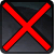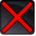Missile BarrageConditions

Related effects, buffs and debuffs

Please click on an effect below to view its details.

• [0s] [not displayed]Effect #1

 Slot: Debuff Duration: 0s Tick rate: does not tick # occurrences: 0
• On Apply

Perform the following actions:

• Add effect #2 to TARGET from TARGET
• [0s] [not displayed]Effect #2

 Slot: Debuff Duration: 0s Tick rate: does not tick # occurrences: 0 Conditions: Can only by called by other effects
• On Apply

Perform the following actions:

• Unknown (183)
- Unknown (303) = (bool) true
- Unknown (306) = (bool) false
- Unknown (430) = (bool) false
- Unknown (437) = (bool) false
- Unknown (310) = (string) aoePositionOptionType_Entity
- Unknown (311) = (string) aoeTarget_Caster
- Unknown (313) = (string) aoeScalarType_LiteralValue
- Unknown (317) = (string) aoeScalarType_LiteralValue
- Unknown (321) = (string) aoeScalarType_LiteralValue
- Unknown (325) = (string) aoeOrientationOptionType_EntityFacing
- Unknown (326) = (string) aoeTarget_Target
- Unknown (344) = (string) aoeCylinder
- Unknown (349) = (string)
- Unknown (353) = (string) aoeScalarType_LiteralValue
- Unknown (354) = (string) aoeScalarType_LiteralValue
- Unknown (355) = (string) aoeScalarType_LiteralValue
- Unknown (367) = (string) aoeScalarType_LiteralValue
- Unknown (375) = (string) aoeScalarType_LiteralValue
- Unknown (384) = (string)
- Unknown (386) = (string) aoeAbilityCaster_Caster
- Unknown (387) = (string) aoeAbilityTarget_Target
- Unknown (389) = (string) flashpoint/corellia/empire/enemy/boss_1/walker_rail_shot
- Unknown (431) = (string) aoeRotation_FollowTargetRotation
- Unknown (432) = (string) aoeTarget_Target
- Unknown (438) = (string) aoeRotation_None
- Unknown (545) = (string) aoeVfxEntity_Caster
- Unknown (546) = (string) vfx_cannon_muzzle
- Unknown (547) = (string) aoeVfxEntity_Target
- Unknown (548) = (string)
- Unknown (552) = (string) aoeVfx_TrackEntity
- Ability Spec = (int) 16141112495118147543
- Unknown (307) = (int) 2
- Unknown (308) = (int) 2
- Tick Rate = (float) 1
- Unknown (304) = (float) 0
- Unknown (305) = (float) 0
- Unknown (314) = (float) 0
- Unknown (318) = (float) 0
- Unknown (322) = (float) 0
- Unknown (362) = (float) 0
- Unknown (363) = (float) 0
- Unknown (364) = (float) 0
- Unknown (368) = (float) 0.5
- Unknown (376) = (float) 0.5
- Unknown (390) = (float) 0
- Unknown (398) = (float) 0.1
- Unknown (435) = (float) 0.5
- Unknown (436) = (float) 0.0001